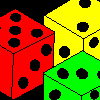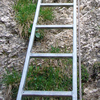# Resources tagged with: Mathematical reasoning & proof

Filter by: Content type:
Age range:
Challenge level:

### There are 91 results

Broad Topics > Mathematical Thinking > Mathematical reasoning & proof### Three Neighbours

##### Age 7 to 11 Challenge Level:

Look at three 'next door neighbours' amongst the counting numbers. Add them together. What do you notice?### What Do You Need?

##### Age 7 to 11 Challenge Level:

Four of these clues are needed to find the chosen number on this grid and four are true but do nothing to help in finding the number. Can you sort out the clues and find the number?##### Age 7 to 14 Challenge Level:

I added together some of my neighbours' house numbers. Can you explain the patterns I noticed?### Even So

##### Age 11 to 14 Challenge Level:

Find some triples of whole numbers a, b and c such that a^2 + b^2 + c^2 is a multiple of 4. Is it necessarily the case that a, b and c must all be even? If so, can you explain why?### What Numbers Can We Make Now?

##### Age 11 to 14 Challenge Level:

Imagine we have four bags containing numbers from a sequence. What numbers can we make now?### Square Subtraction

##### Age 7 to 11 Challenge Level:

Look at what happens when you take a number, square it and subtract your answer. What kind of number do you get? Can you prove it?### What Numbers Can We Make?

##### Age 11 to 14 Challenge Level:

Imagine we have four bags containing a large number of 1s, 4s, 7s and 10s. What numbers can we make?##### Age 11 to 14 Challenge Level:

Powers of numbers behave in surprising ways. Take a look at some of these and try to explain why they are true.### One O Five

##### Age 11 to 14 Challenge Level:

You can work out the number someone else is thinking of as follows. Ask a friend to think of any natural number less than 100. Then ask them to tell you the remainders when this number is divided by. . . .### Elevenses

##### Age 11 to 14 Challenge Level:

How many pairs of numbers can you find that add up to a multiple of 11? Do you notice anything interesting about your results?##### Age 11 to 14 Challenge Level:

Make a set of numbers that use all the digits from 1 to 9, once and once only. Add them up. The result is divisible by 9. Add each of the digits in the new number. What is their sum? Now try some. . . .### Cycle It

##### Age 11 to 14 Challenge Level:

Carry out cyclic permutations of nine digit numbers containing the digits from 1 to 9 (until you get back to the first number). Prove that whatever number you choose, they will add to the same total.### Cows and Sheep

##### Age 7 to 11 Challenge Level:

Use your logical reasoning to work out how many cows and how many sheep there are in each field.### Take Three Numbers

##### Age 7 to 11 Challenge Level:

What happens when you add three numbers together? Will your answer be odd or even? How do you know?##### Age 5 to 11 Challenge Level:

Who said that adding couldn't be fun?### Unit Fractions

##### Age 11 to 14 Challenge Level:

Consider the equation 1/a + 1/b + 1/c = 1 where a, b and c are natural numbers and 0 < a < b < c. Prove that there is only one set of values which satisfy this equation.### Making Pathways

##### Age 7 to 11 Challenge Level:

Can you find different ways of creating paths using these paving slabs?### Online

##### Age 7 to 11 Challenge Level:

A game for 2 players that can be played online. Players take it in turns to select a word from the 9 words given. The aim is to select all the occurrences of the same letter.### Eleven

##### Age 11 to 14 Challenge Level:

Replace each letter with a digit to make this addition correct.### Take One Example

##### Age 5 to 11

This article introduces the idea of generic proof for younger children and illustrates how one example can offer a proof of a general result through unpacking its underlying structure.### Aba

##### Age 11 to 14 Challenge Level:

In the following sum the letters A, B, C, D, E and F stand for six distinct digits. Find all the ways of replacing the letters with digits so that the arithmetic is correct.### Tis Unique

##### Age 11 to 14 Challenge Level:

This addition sum uses all ten digits 0, 1, 2...9 exactly once. Find the sum and show that the one you give is the only possibility.### Chocolate Maths

##### Age 11 to 14 Challenge Level:

Pick the number of times a week that you eat chocolate. This number must be more than one but less than ten. Multiply this number by 2. Add 5 (for Sunday). Multiply by 50... Can you explain why it. . . .### Always the Same

##### Age 11 to 14 Challenge Level:

Arrange the numbers 1 to 16 into a 4 by 4 array. Choose a number. Cross out the numbers on the same row and column. Repeat this process. Add up you four numbers. Why do they always add up to 34?### Always, Sometimes or Never? Number

##### Age 7 to 11 Challenge Level:

Are these statements always true, sometimes true or never true?### Always, Sometimes or Never?

##### Age 5 to 11 Challenge Level:

Are these statements relating to odd and even numbers always true, sometimes true or never true?### Not Necessarily in That Order

##### Age 11 to 14 Challenge Level:

Baker, Cooper, Jones and Smith are four people whose occupations are teacher, welder, mechanic and programmer, but not necessarily in that order. What is each person’s occupation?### More Number Pyramids

##### Age 11 to 14 Challenge Level:

When number pyramids have a sequence on the bottom layer, some interesting patterns emerge...### The Triangle Game

##### Age 11 to 16 Challenge Level:

Can you discover whether this is a fair game?### Pyramids

##### Age 11 to 14 Challenge Level:

What are the missing numbers in the pyramids?### Go Forth and Generalise

##### Age 11 to 14

Spotting patterns can be an important first step - explaining why it is appropriate to generalise is the next step, and often the most interesting and important.### More Marbles

##### Age 11 to 14 Challenge Level:

I start with a red, a blue, a green and a yellow marble. I can trade any of my marbles for three others, one of each colour. Can I end up with exactly two marbles of each colour?### Marbles

##### Age 11 to 14 Challenge Level:

I start with a red, a green and a blue marble. I can trade any of my marbles for two others, one of each colour. Can I end up with five more blue marbles than red after a number of such trades?### Tri-colour

##### Age 11 to 14 Challenge Level:

Six points are arranged in space so that no three are collinear. How many line segments can be formed by joining the points in pairs?### Disappearing Square

##### Age 11 to 14 Challenge Level:

Do you know how to find the area of a triangle? You can count the squares. What happens if we turn the triangle on end? Press the button and see. Try counting the number of units in the triangle now. . . .### Children at Large

##### Age 11 to 14 Challenge Level:

There are four children in a family, two girls, Kate and Sally, and two boys, Tom and Ben. How old are the children?### Tower of Hanoi

##### Age 11 to 14 Challenge Level:

The Tower of Hanoi is an ancient mathematical challenge. Working on the building blocks may help you to explain the patterns you notice.### Sticky Numbers

##### Age 11 to 14 Challenge Level:

Can you arrange the numbers 1 to 17 in a row so that each adjacent pair adds up to a square number?### Dicing with Numbers

##### Age 11 to 14 Challenge Level:

In how many ways can you arrange three dice side by side on a surface so that the sum of the numbers on each of the four faces (top, bottom, front and back) is equal?### More Mathematical Mysteries

##### Age 11 to 14 Challenge Level:

Write down a three-digit number Change the order of the digits to get a different number Find the difference between the two three digit numbers Follow the rest of the instructions then try. . . .### 9 Weights

##### Age 11 to 14 Challenge Level:

You have been given nine weights, one of which is slightly heavier than the rest. Can you work out which weight is heavier in just two weighings of the balance?##### Age 7 to 11 Challenge Level:

Three dice are placed in a row. Find a way to turn each one so that the three numbers on top of the dice total the same as the three numbers on the front of the dice. Can you find all the ways to do. . . .##### Age 11 to 14 Challenge Level:

A little bit of algebra explains this 'magic'. Ask a friend to pick 3 consecutive numbers and to tell you a multiple of 3. Then ask them to add the four numbers and multiply by 67, and to tell you. . . .### Always, Sometimes or Never? Shape

##### Age 7 to 11 Challenge Level:

Are these statements always true, sometimes true or never true?### Logic

##### Age 7 to 14

What does logic mean to us and is that different to mathematical logic? We will explore these questions in this article.### Reasoning: the Journey from Novice to Expert (article)

##### Age 5 to 11

This article for primary teachers suggests ways in which we can help learners move from being novice reasoners to expert reasoners.### Con Tricks

##### Age 11 to 14

Here are some examples of 'cons', and see if you can figure out where the trick is.### Konigsberg Plus

##### Age 11 to 14 Challenge Level:

Euler discussed whether or not it was possible to stroll around Koenigsberg crossing each of its seven bridges exactly once. Experiment with different numbers of islands and bridges.### Tourism

##### Age 11 to 14 Challenge Level:

If you can copy a network without lifting your pen off the paper and without drawing any line twice, then it is traversable. Decide which of these diagrams are traversable.### Sprouts Explained

##### Age 7 to 18

This article invites you to get familiar with a strategic game called "sprouts". The game is simple enough for younger children to understand, and has also provided experienced mathematicians with. . . .